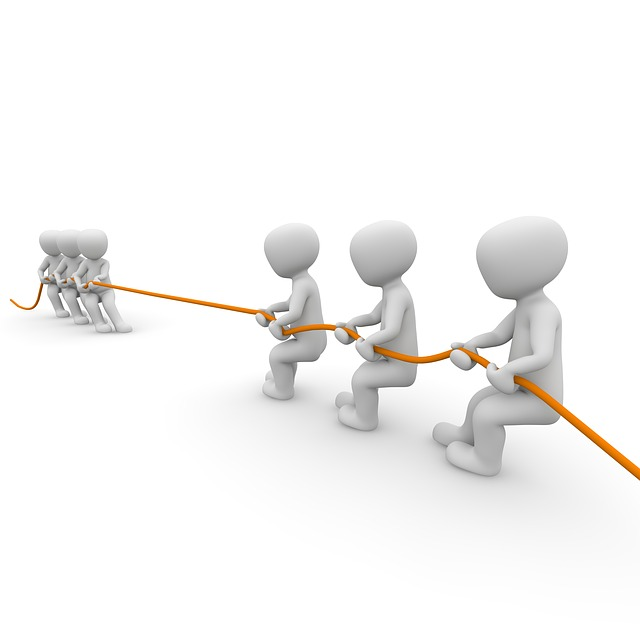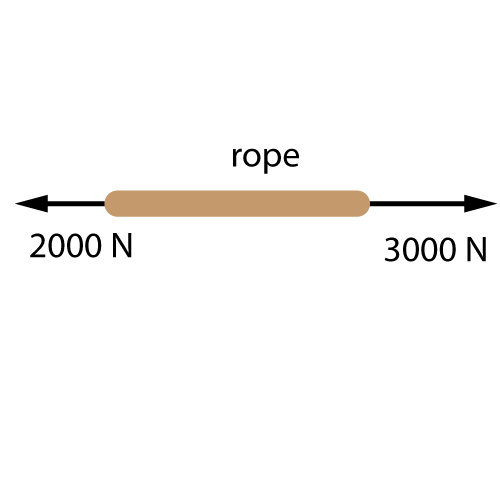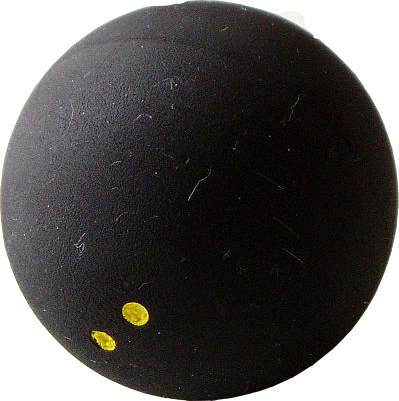µ GCSE | Balancing Forces

10 quick questions - for GCSE and iGCSE

10 minutes maximum! (can you do it in 5?)

1. Two teams are pulling on a rope. (A 'tug-of-war'). The arrows show the sizes of the forces.The total force ('resultant force' is)

• A. zero - the forces are balanced.
• B. 1000 N to the left.
• C. 1000 N to the right.
• D. 5000 N to the right.

2. Which of these is NOT a type of force?

• A. Magnetism
• B. Air resistance
• C. Velocity
• D. Friction

3. An unbalanced force on a squash ball can do many things, but it CANNOT...

• A. change the mass of the ball.
• B. change the speed of the ball.
• C. change the shape of the ball.
• D. change the direction of motion of the ball.

A squash ball is dropped from a tall building .

Q 4-8. What are the missing words in this passage?As the ball accelerates, the downwards force of gravity on it ? increases decreases stays the same changes direction , whilst the air resistance ? increases decreases stays the same changes direction . Eventually the two forces are ? stationary balanced unbalanced . The resultant force on the ball is now ? zero upwards downwards . This means it stays at a constant speed, called the ? gravitational field strength terminal velocity maximum resistance reaction force .

9. Which of these graphs shows the velocity of the squash ball as it falls?10. A battery powered toy car has many forces acting on it when moving across the floor. Which of these diagrams show the correct sized arrows for the car accelerating to the right?